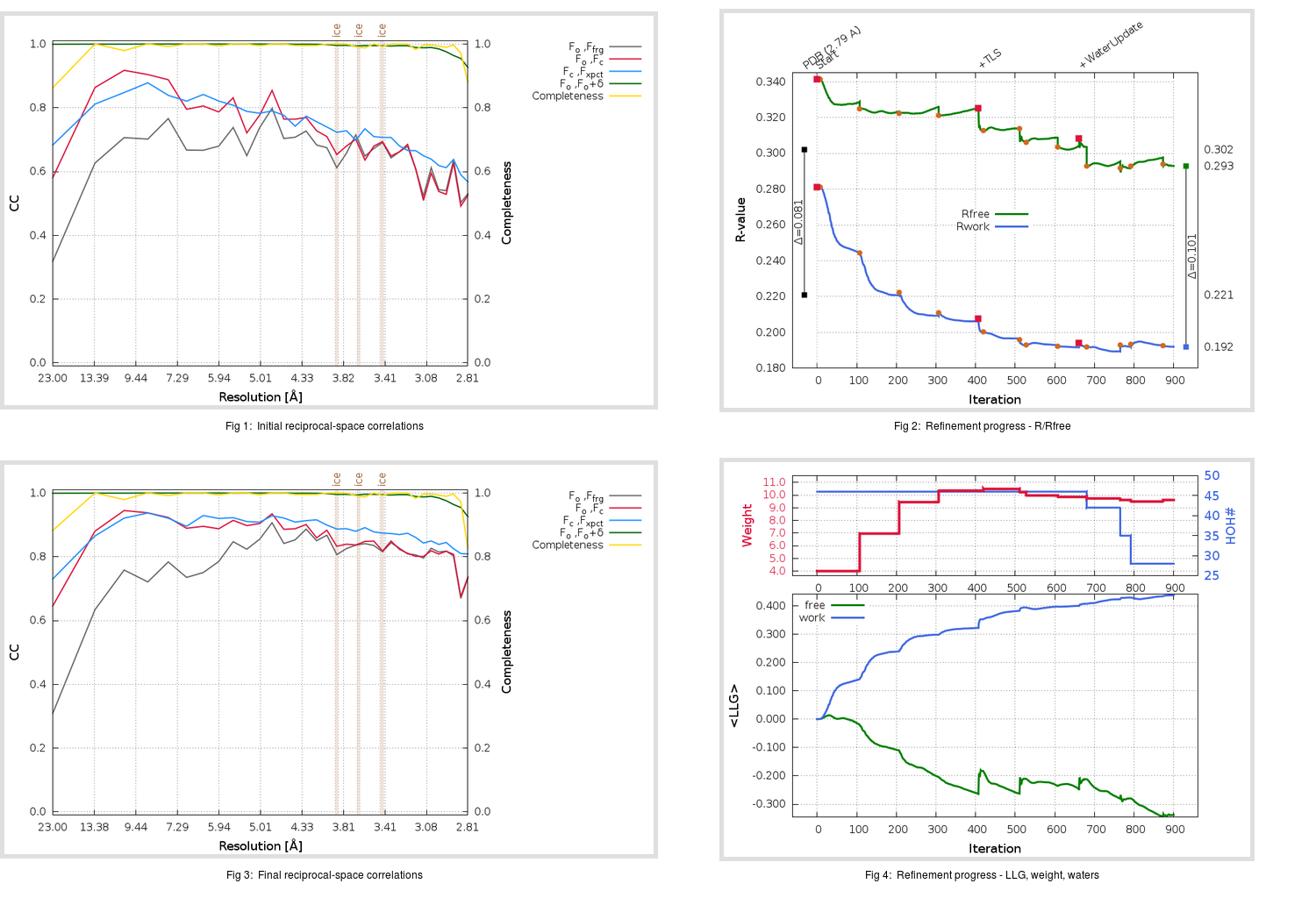Content:

```    Diffraction limits & principal axes of ellipsoid fitted to diffraction cut-off surface:
2.739         1.0000   0.0000   0.0000       a*
2.689         0.0000   1.0000   0.0000       b*
2.706         0.0000   0.0000   1.0000       c*
```

## Deposited

` `
 Date deposited Date data collection Resolution R, Rfree 20050722 20050620 2.79 0.2080 0.3020

Molprobity (CCP4 7.0 version) summary:

```Ramachandran outliers =   1.35 %
favored =  92.91 %
Rotamer outliers      =   4.28 %
C-beta deviations     =     0
Clashscore            =   2.18
RMS(bonds)            =   0.0152
RMS(angles)           =   1.33
MolProbity score      =   1.92
Resolution            =   2.79
R-work                =   0.2080
R-free                =   0.3020
```

```Number of waters      =    46

<B> (all atoms) =   56.14 ( sd =    6.25 ) for       2362 non-hydrogen atoms
<B>   (protein) =   56.14 ( sd =    6.10 ) for       2316 non-hydrogen atoms
<B>     (water) =   55.77 ( sd =   11.72 ) for         46 non-hydrogen atoms
<B>    (others) =    0.00 ( sd =    0.00 ) for          0 non-hydrogen atoms

B min/max       (all non-hydrogen atoms) =   28.29 /   76.79
B min/max   (protein non-hydrogen atoms) =   46.04 /   74.91
B min/max     (water non-hydrogen atoms) =   28.29 /   76.79
B min/max     (other non-hydrogen atoms) =    0.00 /    0.00
```

## BUSTER (re-)refinement

` `

Molprobity (CCP4 7.0 version) summary:

```Ramachandran outliers =   2.03 %
favored =  90.54 %
Rotamer outliers      =  10.12 %
C-beta deviations     =     1
Clashscore            =   7.85
RMS(bonds)            =   0.0118
RMS(angles)           =   1.62
MolProbity score      =   2.73
Resolution            =   2.79
R-work                =   0.1920
R-free                =   0.2930
```

```Number of waters      =    28

<B> (all atoms) =   71.10 ( sd =   16.10 ) for       2344 non-hydrogen atoms
<B>   (protein) =   71.20 ( sd =   16.08 ) for       2316 non-hydrogen atoms
<B>     (water) =   62.45 ( sd =   14.40 ) for         28 non-hydrogen atoms
<B>    (others) =    0.00 ( sd =    0.00 ) for          0 non-hydrogen atoms

B min/max       (all non-hydrogen atoms) =   38.39 /  120.46
B min/max   (protein non-hydrogen atoms) =   38.39 /  120.46
B min/max     (water non-hydrogen atoms) =   44.57 /  106.43
B min/max     (other non-hydrogen atoms) =    0.00 /    0.00
```

Refinement progression:Results:

` `
 File Remark 2BX4_aB_refine.01_03_refine.pdb.gz exact refinement commands are in header 2BX4_aB_refine.01_03_refine.mtz.gz including original deposited data and several re-refinement map coefficients 2BX4_aB_refine.01_03_BUSTER_model.cif.gz including any non-standard compound restraints 2BX4_aB_refine.01_03_BUSTER_refln.cif.gz